3.3 LRUCache

# 最近最少使用 LRUCache #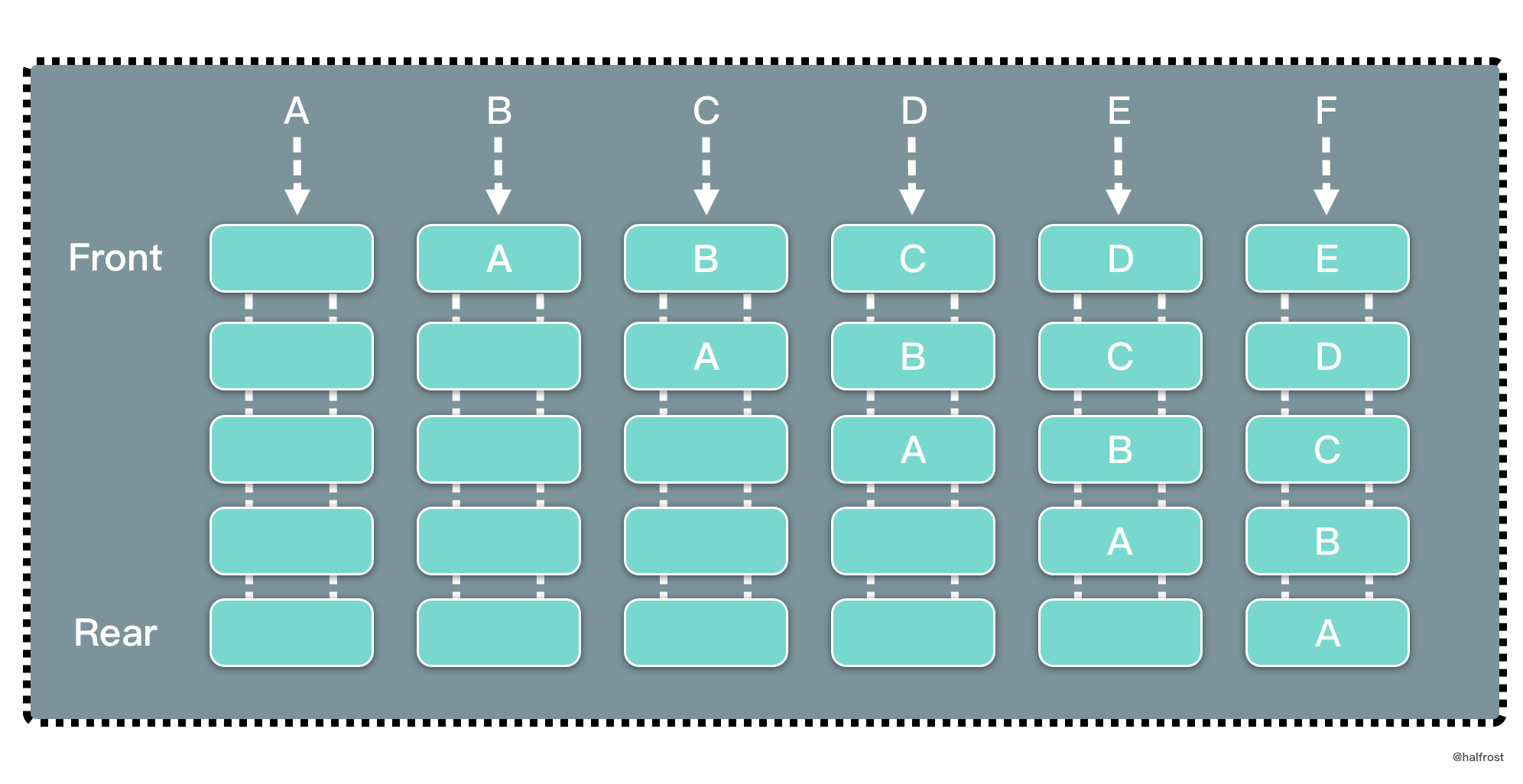LRU 是 Least Recently Used 的缩写，即最近最少使用，是一种常用的页面置换算法，选择最近最久未使用的页面予以淘汰。如上图，要插入 F 的时候，此时需要淘汰掉原来的一个页面。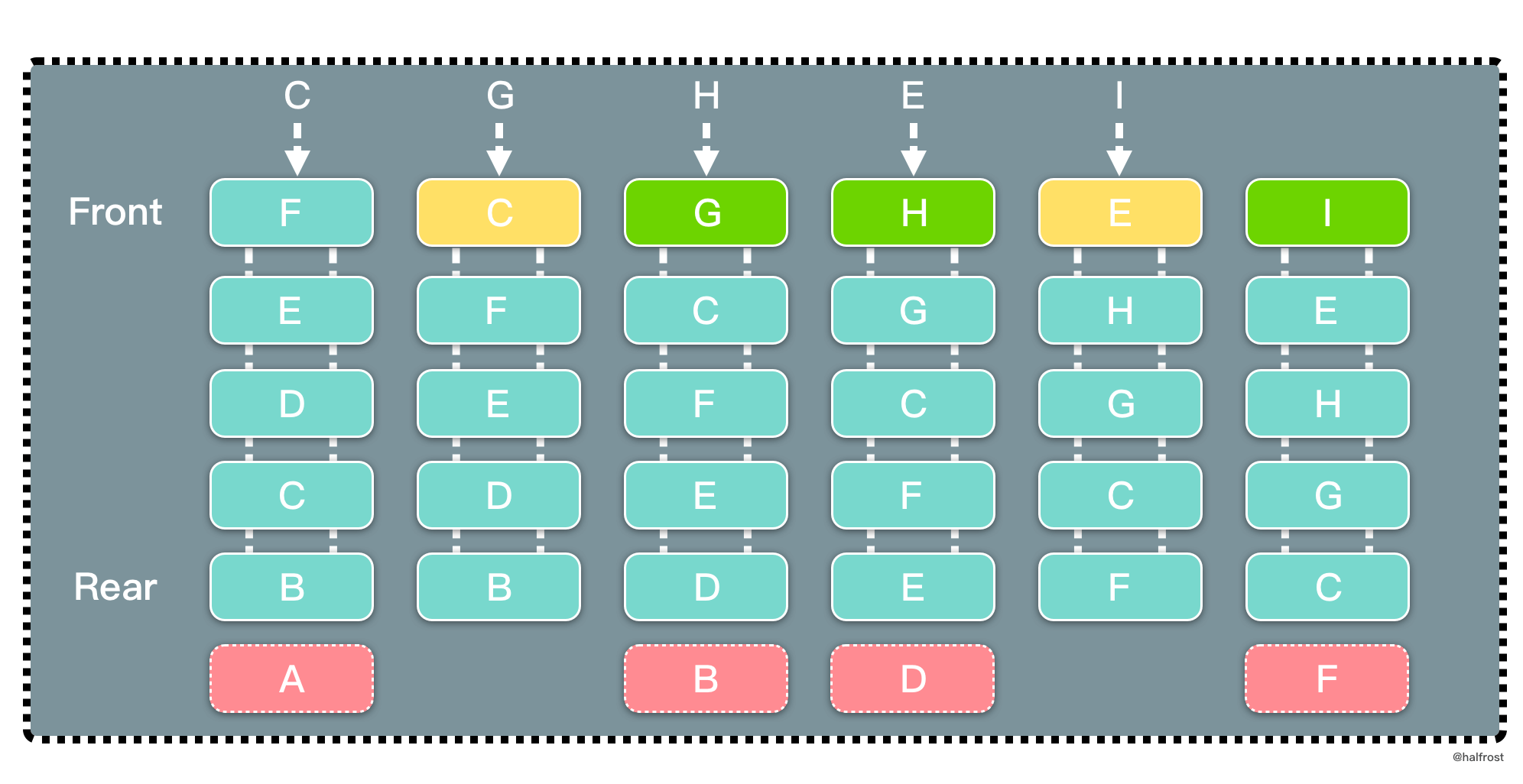## 解法一 Get O(1) / Put O(1) #

LRU 要求查询尽量高效，O(1) 内查询。那肯定选用 map 查询。修改，删除也要尽量 O(1) 完成。搜寻常见的数据结构，链表，栈，队列，树，图。树和图排除，栈和队列无法任意查询中间的元素，也排除。所以选用链表来实现。但是如果选用单链表，删除这个结点，需要 O(n) 遍历一遍找到前驱结点。所以选用双向链表，在删除的时候也能 O(1) 完成。

``````import "container/list"

type LRUCache struct {
Cap  int
Keys map[int]*list.Element
List *list.List
}

type pair struct {
K, V int
}

func Constructor(capacity int) LRUCache {
return LRUCache{
Cap: capacity,
Keys: make(map[int]*list.Element),
List: list.New(),
}
}

``````

``````type Element struct {
// Next and previous pointers in the doubly-linked list of elements.
// To simplify the implementation, internally a list l is implemented
// as a ring, such that &l.root is both the next element of the last
// list element (l.Back()) and the previous element of the first list
// element (l.Front()).
next, prev *Element

// The list to which this element belongs.
list *List

// The value stored with this element.
Value interface{}
}
``````

LRUCache 的 Get 操作很简单，在 map 中直接读取双向链表的结点。如果 map 中存在，将它移动到双向链表的表头，并返回它的 value 值，如果 map 中不存在，返回 -1。

``````func (c *LRUCache) Get(key int) int {
if el, ok := c.Keys[key]; ok {
c.List.MoveToFront(el)
return el.Value.(pair).V
}
return -1
}
``````

LRUCache 的 Put 操作也不难。先查询 map 中是否存在 key，如果存在，更新它的 value，并且把该结点移到双向链表的表头。如果 map 中不存在，新建这个结点加入到双向链表和 map 中。最后别忘记还需要维护双向链表的 cap，如果超过 cap，需要淘汰最后一个结点，双向链表中删除这个结点，map 中删掉这个结点对应的 key。

``````func (c *LRUCache) Put(key int, value int) {
if el, ok := c.Keys[key]; ok {
el.Value = pair{K: key, V: value}
c.List.MoveToFront(el)
} else {
el := c.List.PushFront(pair{K: key, V: value})
c.Keys[key] = el
}
if c.List.Len() > c.Cap {
el := c.List.Back()
c.List.Remove(el)
delete(c.Keys, el.Value.(pair).K)
}
}

``````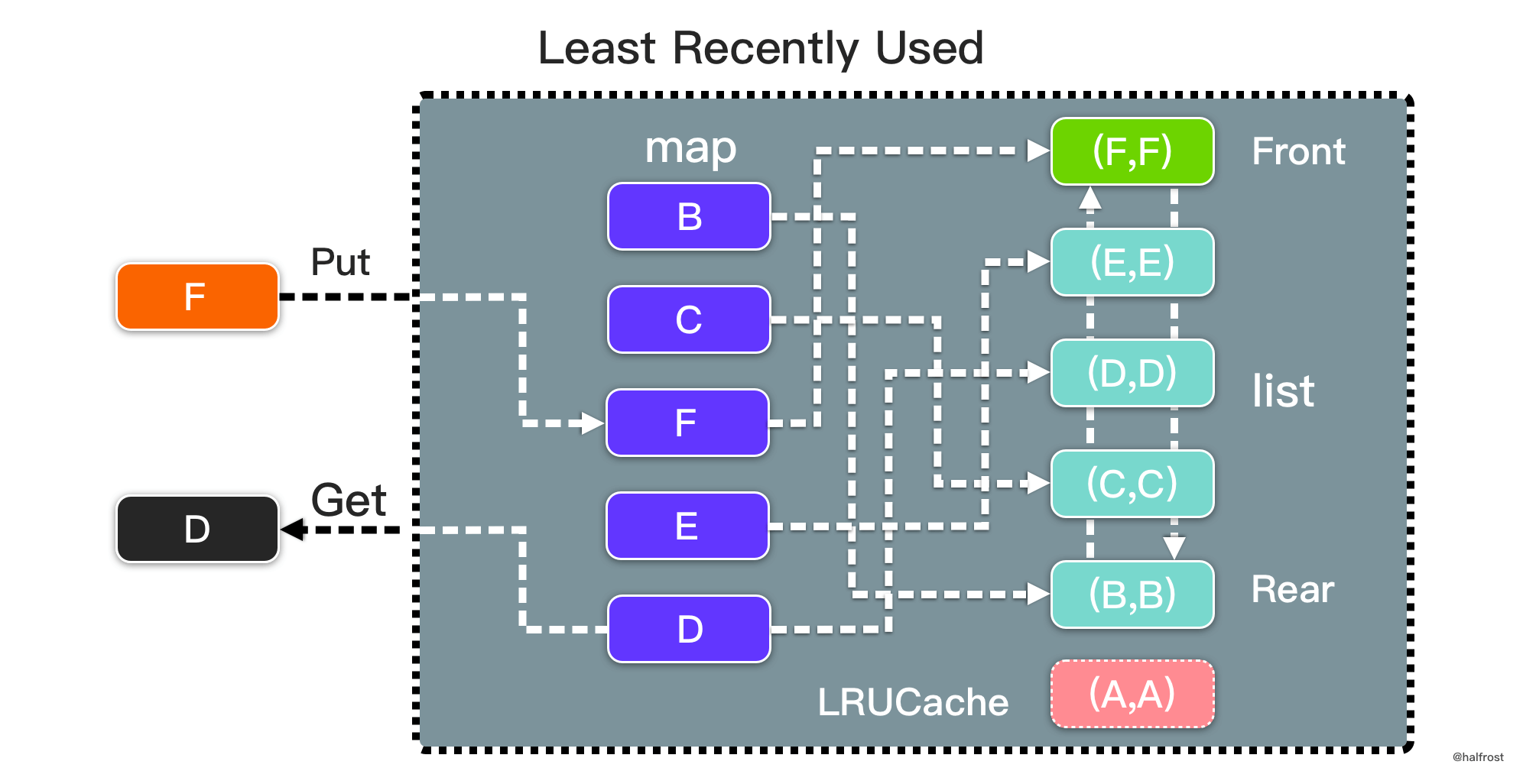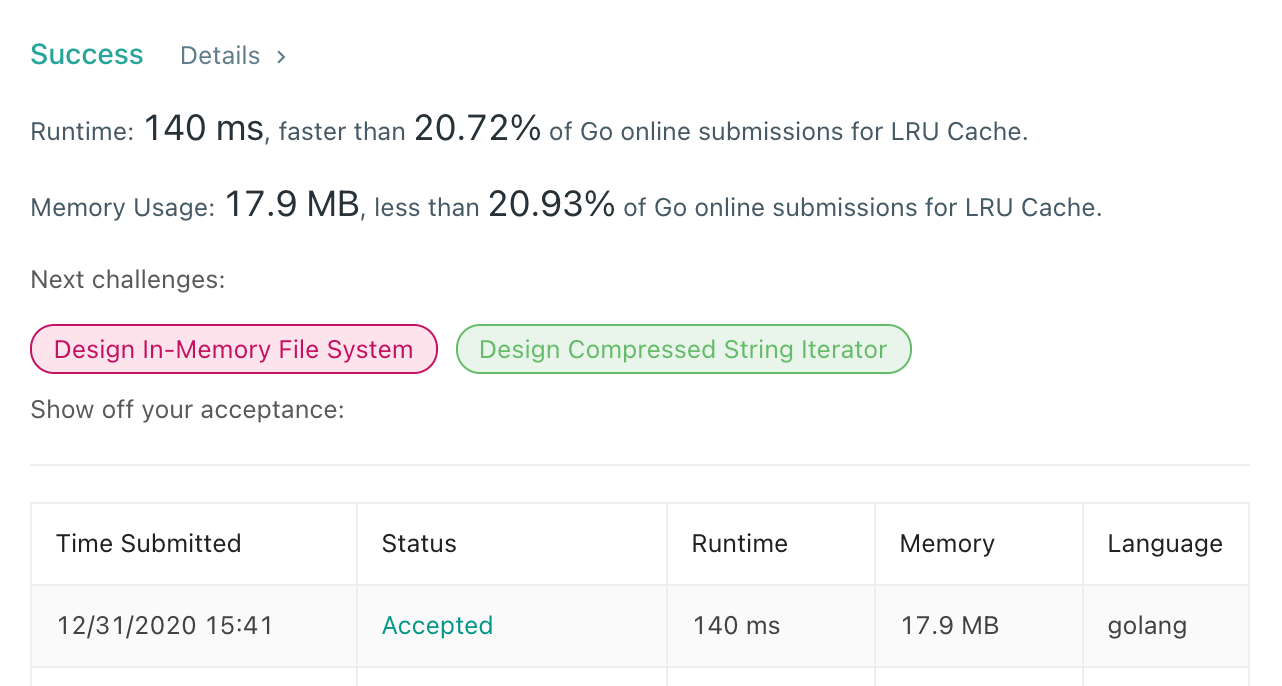## 解法二 Get O(1) / Put O(1) #

``````
type LRUCache struct {
keys       map[int]*Node
capacity   int
}

type Node struct {
key, val   int
prev, next *Node
}

func ConstructorLRU(capacity int) LRUCache {
return LRUCache{keys: make(map[int]*Node), capacity: capacity}
}

func (this *LRUCache) Get(key int) int {
if node, ok := this.keys[key]; ok {
this.Remove(node)
return node.val
}
return -1
}

func (this *LRUCache) Put(key int, value int) {
if node, ok := this.keys[key]; ok {
node.val = value
this.Remove(node)
return
} else {
node = &Node{key: key, val: value}
this.keys[key] = node
}
if len(this.keys) > this.capacity {
delete(this.keys, this.tail.key)
this.Remove(this.tail)
}
}

func (this *LRUCache) Add(node *Node) {
node.prev = nil
}
if this.tail == nil {
this.tail = node
this.tail.next = nil
}
}

func (this *LRUCache) Remove(node *Node) {
if node.next != nil {
node.next.prev = nil
}
node.next = nil
return
}
if node == this.tail {
this.tail = node.prev
node.prev.next = nil
node.prev = nil
return
}
node.prev.next = node.next
node.next.prev = node.prev
}

``````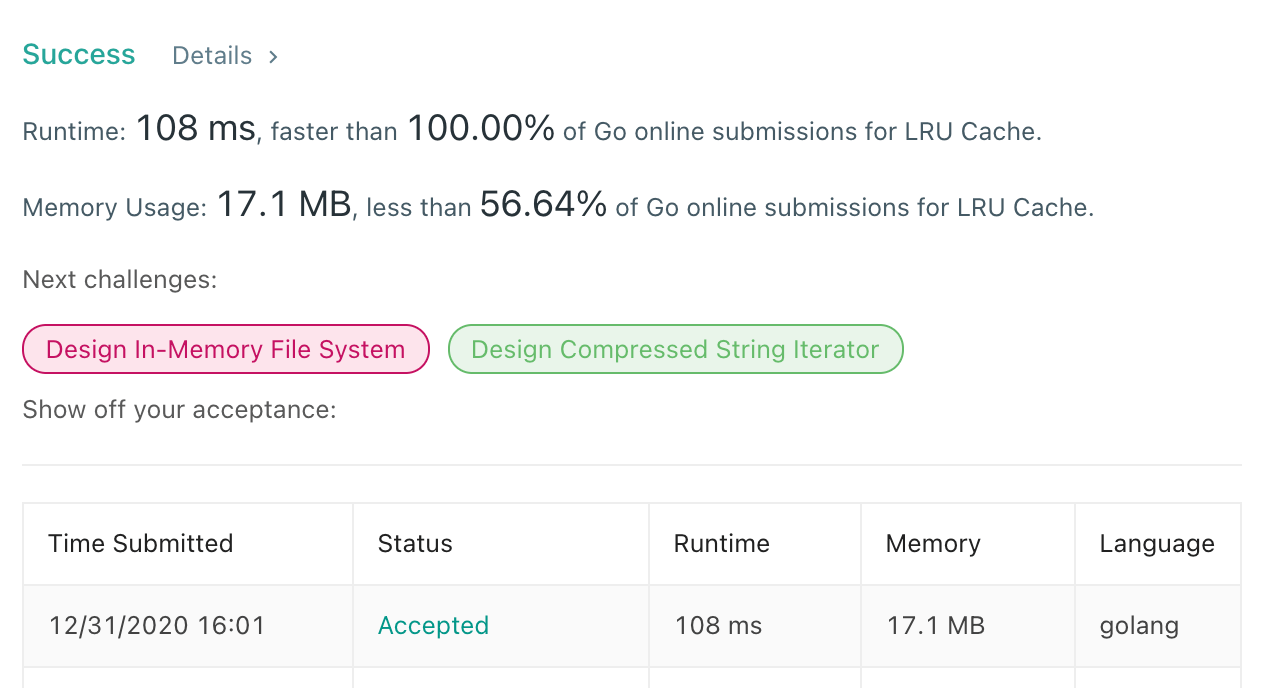## 模板 #

``````type LRUCache struct {
Keys       map[int]*Node
Cap        int
}

type Node struct {
Key, Val   int
Prev, Next *Node
}

func Constructor(capacity int) LRUCache {
return LRUCache{Keys: make(map[int]*Node), Cap: capacity}
}

func (this *LRUCache) Get(key int) int {
if node, ok := this.Keys[key]; ok {
this.Remove(node)
return node.Val
}
return -1
}

func (this *LRUCache) Put(key int, value int) {
if node, ok := this.Keys[key]; ok {
node.Val = value
this.Remove(node)
return
} else {
node = &Node{Key: key, Val: value}
this.Keys[key] = node
}
if len(this.Keys) > this.Cap {
delete(this.Keys, this.tail.Key)
this.Remove(this.tail)
}
}

func (this *LRUCache) Add(node *Node) {
node.Prev = nil
}
if this.tail == nil {
this.tail = node
this.tail.Next = nil
}
}

func (this *LRUCache) Remove(node *Node) {
node.Next = nil
return
}
if node == this.tail {
this.tail = node.Prev
node.Prev.Next = nil
node.Prev = nil
return
}
node.Prev.Next = node.Next
node.Next.Prev = node.Prev
}

``````Apr 8, 2023Edit this page